Question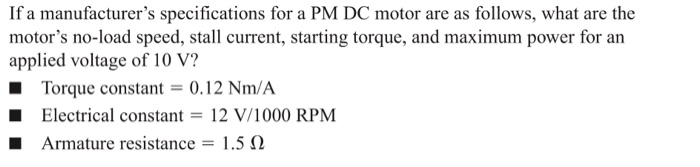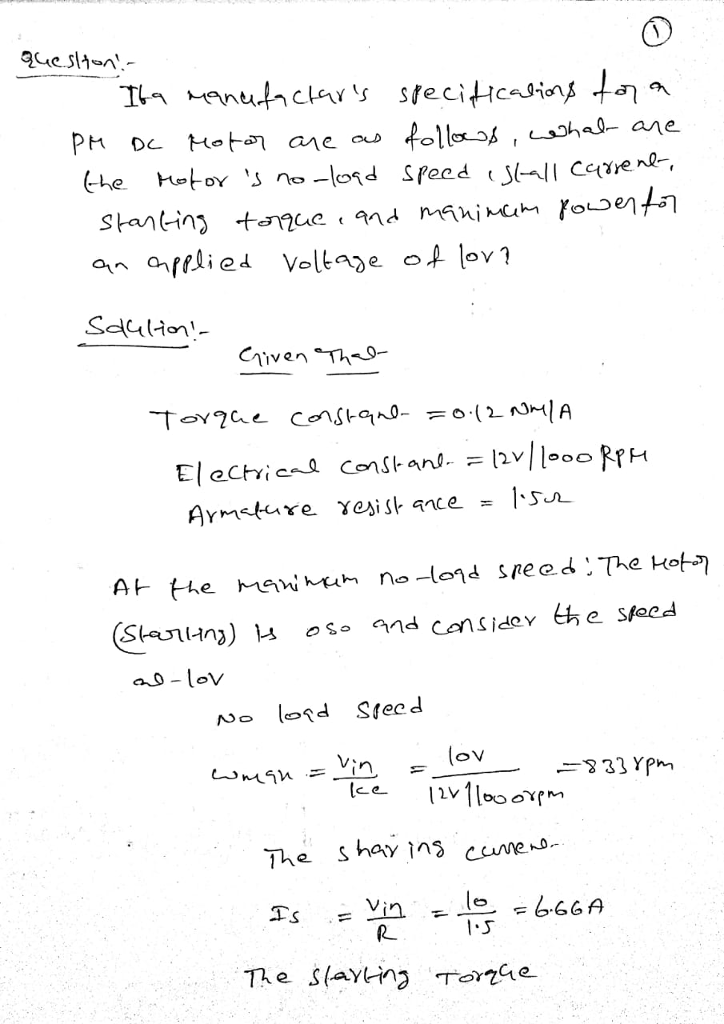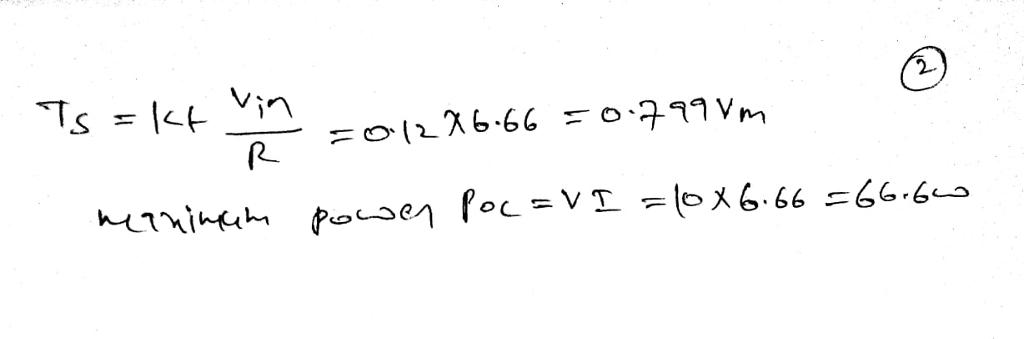#### Earn Coins

Coins can be redeemed for fabulous gifts.

Similar Homework Help Questions
• ### An armature-controlled dc motor operates at steady state, with an armature drive voltage va = 10 ...

An armature-controlled dc motor operates at steady state, with an armature drive voltage va = 10 V. The motor runs at 600 rpm, and the armature current is found to be ia = 0.2 A. The armature resistance is Ra = 15 Ω. Determine: (a) The torque constant km of the motor (b) Electrical damping constant be (c) The efficiency under the given operating conditions, if the mechanical damping constant is bm = 8.25 × 10−5 N ⋅ m/rad/s (d)...

• ### Question 3: DC motors can be simplistically modeled as shown in Figure 5 MoTor back emf Vi: +1 Re...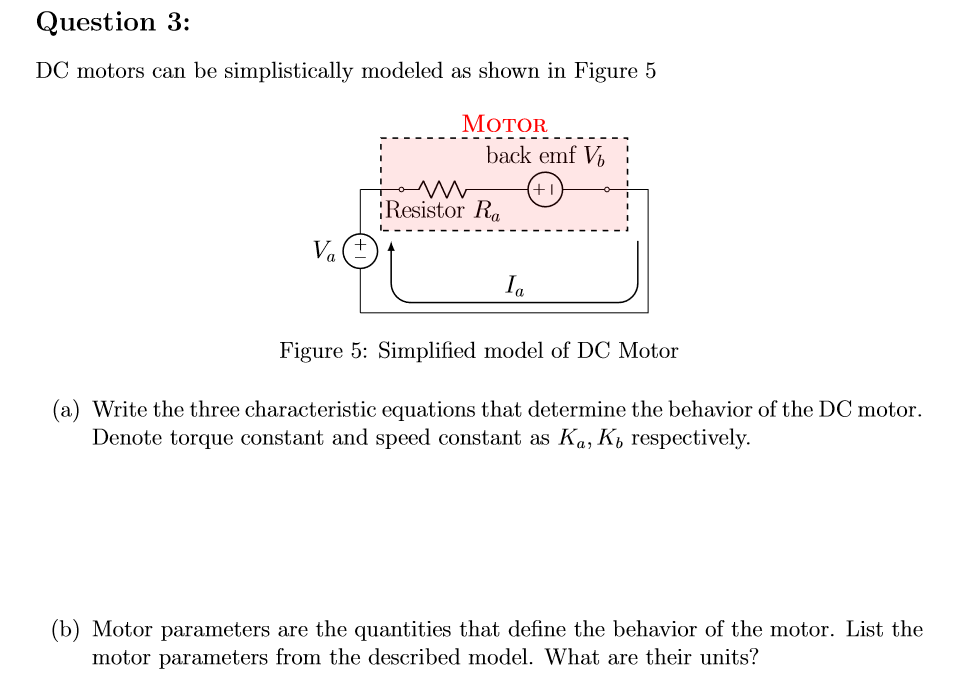Question 3: DC motors can be simplistically modeled as shown in Figure 5 MoTor back emf Vi: +1 Resistor Ra Ia Figure 5: Simplified model of DC Motor (a) Write the three characteristic equations that determine the behavior of the DC motor. Denote torque constant and speed constant as Ka, K, respectively. (b) Motor parameters are the quantities that define the behavior of the motor. List the motor parameters from the described model. What are their units? (c) For given...

• ### Open Book Exam A 220 V, 1000 V dc-sourc rpm, separately dc motor is fed from a dc-dc converter th...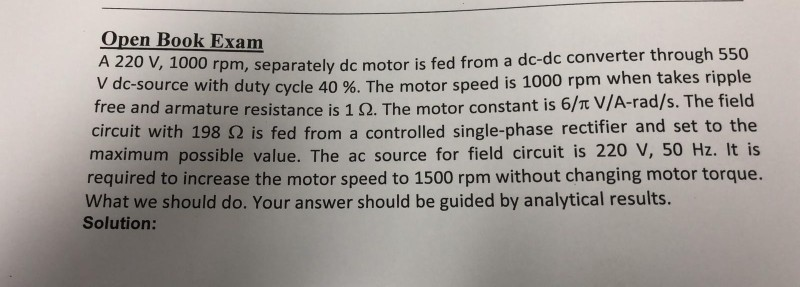Open Book Exam A 220 V, 1000 V dc-sourc rpm, separately dc motor is fed from a dc-dc converter through 550 e with duty cycle 40 %. The motor speed is 1000 rpm when takes ripple free and armature resistance is 1 Ω. The motor constant is 6/π V/A-rad/s. The field circuit with 198 Ω is fed from a controlled single-phase rectifier and set to the maximum possible value. The ac source for field circuit is 220 V, 50 Hz....

• ### The permanent DC motor model CDP3450 by ABB is rated at 1 Hp, 2 500 RMP...

The permanent DC motor model CDP3450 by ABB is rated at 1 Hp, 2 500 RMP at 90v (no load). The armature resistance is 0.425 Ohm. a. The continuous current rating of this motor is 9.7 Amp, what is the maximum continuous torque rating? b. The motor is to drive a load of 2NM torque while maintaining a constant speed of 2000 RPM. What is the required supply current and voltage? c. With 90v armature voltage, what is the maximum...

• ### need the answer for the question no. 1 ENGR 113 Homework DC Motor Objectives Plot the...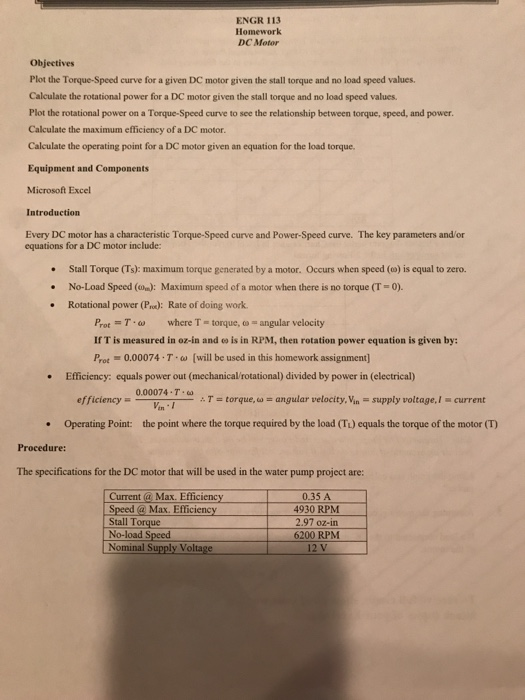need the answer for the question no. 1 ENGR 113 Homework DC Motor Objectives Plot the Torque-Speed curve for a given DC motor given the stall torque and no load speed values Calculate the rotational power for a DC motor given the stall torque and no load speed values Plot the rotational power on a Torque-Speed curve to see the relationship between torque, speed, and power Calculate the maximum efficiency of a DC motor. Calculate the operating point for a...

• ### Q1b) DC Machines [ 6 marks ] You are given a 24 V DC motor. When...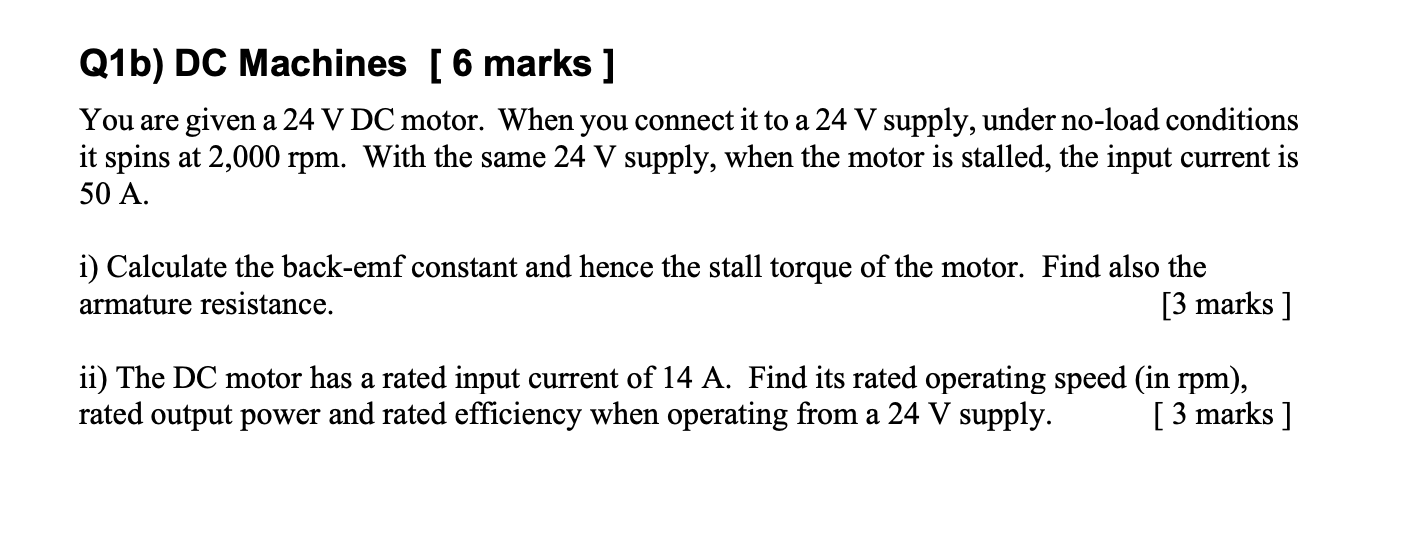Q1b) DC Machines [ 6 marks ] You are given a 24 V DC motor. When you connect it to a 24 V supply, under no-load conditions it spins at 2,000 rpm. With the same 24 V supply, when the motor is stalled, the input current is 50 A. i) Calculate the back-emf constant and hence the stall torque of the motor. Find also the armature resistance. [3 marks ] ii) The DC motor has a rated input current of...

• ### A motor drives a load requiring 0.006 N-m. Motor data is as follows: armature resistance, 4...

A motor drives a load requiring 0.006 N-m. Motor data is as follows: armature resistance, 4 ohms; applied voltage, 8 volts; torque constant (KT), 0.06 N-m/A; generating constant (KE), 0.0026 volts/rpm. Find the motor's running speed.

• ### A separately excited DC motor has the following data P=32kW (rated power); Va=220V (rated voltage); Ia=171A...

A separately excited DC motor has the following data P=32kW (rated power); Va=220V (rated voltage); Ia=171A (rated curent); n=1000 rpm (rated speed); Ra = 0.096 ohm a.Calculate the armature voltage to reduce the speed to 900 rpm, assuming the load torque is kept constant? b.Calculate the resistance should be added to armature circuit to reduce the speed to 800 rpm, assuming the load torque is kept constant? c.Calculate the reduction of magnetic flux to reach the speed of 1100 rpm,...

• ### Problem 2 A separately excited DC motor is characterized by the followng intormation Armature res...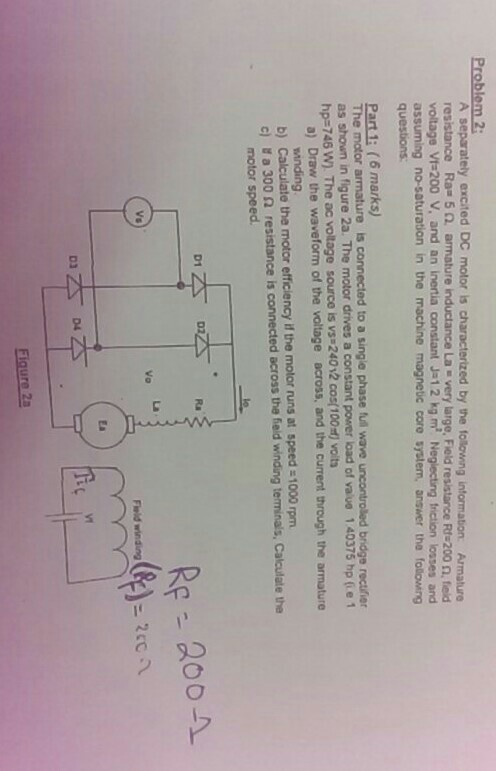Problem 2 A separately excited DC motor is characterized by the followng intormation Armature resistance Ra-5 2 armature inductance La . very large, Field resistance RS200 Ω, ted voltage Vt 200 V, and an inertia constant J-12 kg m Neglecting friction losses and assuming no-saturation in the machine magnetic core system, answer the folowing questions: Part 1: (6 marks) he motor a as shown in figure 2a. The motor drives a constant power load of vaue 1 40375 hp (ie...

• ### A 6 hp, 180 V shunt dc motor draws a full-load current of 30 A from the power supply. The motor h...

A 6 hp, 180 V shunt dc motor draws a full-load current of 30 A from the power supply. The motor has a field (shunt) resistance of 150 Ω. The armature resistance of the motor is 0.1 Ω. When the motor speed is 1800 rpm (this is not the full-load speed), the relationship between the induced voltage